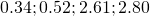# Solution assignment 10 Trigonometric equations

### Assignment 10

Solve for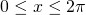: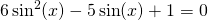### Solution

We substitute: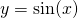in the equation and we get: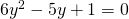This is a quadratic equation and the solutions are: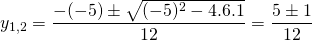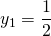or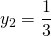Now we have to solve the equations: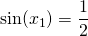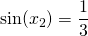The first equation yields: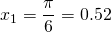or: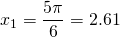The second solution can be calculated using a calculator. We find approximately: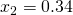or: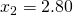Thus the equation has four solutions in the given interval: